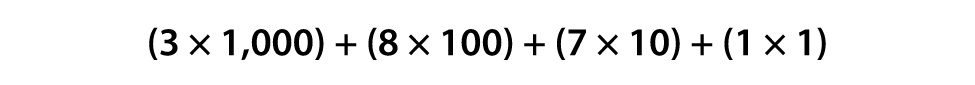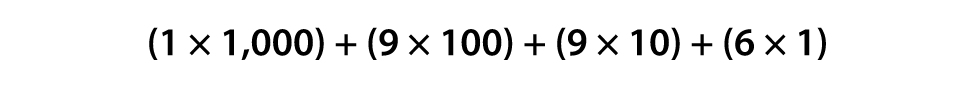# Lesson 1: Numbers to 10,000 Review

## Getting Started

### Questions to Explore

• How does place value work?
• How can we use place value to name, create, and compare numbers to a million?
• How do we write and speak in mathematical language?

### Facts and Definitions

• Ones place: position on the right in a number
• Tens place: position to the left of the ones place
• Hundreds place: position to the left of the tens place
• Thousands place: position to the left of the hundreds place
• Expanded form: a way to write a number that shows the value of each digit (for example, 4,529=4000+500+20+9)
• Expanded notation: also called super expanded form, expresses a number by showing each digit multiplied by its place value. For example, 4,532 in expanded notation is (4×1,000)+(5×100)+(3×10)+(2×1)

### Skills

• Read and write multi-digit whole numbers using base-ten numerals, number names, and expanded form
• Order and compare multi-digit numbers

### Materials

• deck of playing cards (kit)
• fine point dry-erase markers (kit)
• index cards (kit)
• Interactive Notebook
• whiteboard (kit)

### Introduction

Materials: fine point dry-erase markers (kit), whiteboard (kit)
Write 6,482 on the whiteboard, and pose the following questions:
• How many digits does this number have? (4)
• How do we say this number? (six thousand four hundred eighty-two — remind your child as needed that we don't say "and" in between the numbers)
• What digit is in the ones place? (2)
• What digit is in the tens place? (8)
• What digit is in the hundreds place? (4)
• What digit is in the thousands place? (6)
• What is the value of 6 in this number? (6,000 — repeat for each digit)
• What number is 10 more than this number? (6,492)
• What number is 10 less than this number? (6,472)
• What number is 100 more than this number? (6,582)
• What number is 100 less than this number? (6,382)
Now, write 3,871 on the whiteboard, and ask your child to say the number aloud. He should say, "Three thousand eight hundred seventy-one." Ask, "Can you recall another way we can show large numbers?" As needed, remind your child that we also use expanded form to show numbers. Tell him to write 3,871 in expanded form on the whiteboard (3,000+800+70+1). Help him as needed by asking him the value of each digit in the number, such as, "What is the value of the 3 in the thousands place?" (3,000) Repeat this process for the numbers 8,902 (8,000+900+2) and 1,996 (1,000+900+90+6).

Tell your child that expanded notation (sometimes called super expanded form) is another way to represent a number. Tell him that it is similar to expanded form but goes one step further to show each digit multiplied by its place value. Show 3,871 in expanded form again on the whiteboard (3,000+800+70+1), and then show him the expanded notation underneath:Tell your child that this method provides a clear way of showing the value of each place in the number. Explain that the parentheses help the numbers stay organized. Ask him to try this new form with 1,996; write out the expanded form as needed (1,000+900+90+6). His answer should be as follows:Without erasing the previous example, show the expanded form for 8,902 (8,000+900+2). Tell your child that in expanded form, the zero isn't included but that it is in expanded notation since expanded notation shows the value of each place. Ask your child to try to write the expanded notation of 8,902. Remind him that he should take each digit and multiply it by its place value. If he gets stuck when he gets to the 0, ask him what place the digit is in (tens place), so he should multiply the digit (0) by 10. The answer is (8×1,000)+(9×100)+(0×10)+(2×1).
NOTE: In Lesson 3, your child will learn about placing commas in numbers. If he already knows how to put commas in 4-digit numbers, he is free to do so in this lesson.